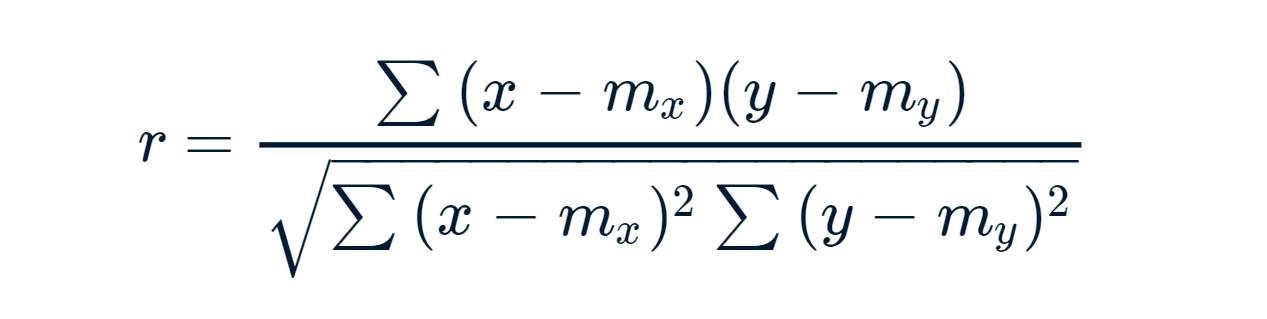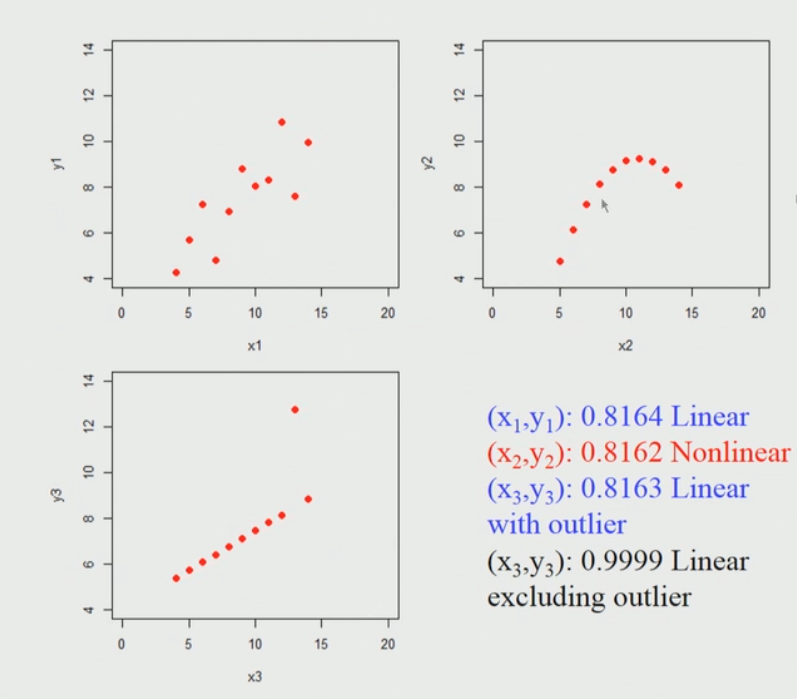Related Articles

# Python – Pearson Correlation Test Between Two Variables

• Last Updated : 15 Sep, 2021

What is correlation test?
The strength of the association between two variables is known as correlation test.
For instance, if we are interested to know whether there is a relationship between the heights of fathers and sons, a correlation coefficient can be calculated to answer this question.
Methods for correlation analyses:

• Parametric Correlation : It measures a linear dependence between two variables (x and y) is known as a parametric correlation test because it depends on the distribution of the data.
• Non-Parametric Correlation: Kendall(tau) and Spearman(rho), which are rank-based correlation coefficients, are known as non-parametric correlation.

Note: The most commonly used method is the Parametric correlation method.
Pearson Correlation formula:x and y are two vectors of length n
m, x and m, y corresponds to the means of x and y, respectively.

Note:

• r takes value between -1 (negative correlation) and 1 (positive correlation).
• r = 0 means no correlation.
• Can not be applied to ordinal variables.
• The sample size should be moderate (20-30) for good estimation.
• Outliers can lead to misleading values means not robust with outliers.

To compute Pearson correlation in Python – pearsonr() function can be used.
Python functions

Syntax:
pearsonr(x, y)
Parameters:
x, y: Numeric vectors with the same length

Code: Python code to find the pearson correlation

## Python3

 `# Import those libraries``import` `pandas as pd``from` `scipy.stats ``import` `pearsonr` `# Import your data into Python``df ``=` `pd.read_csv(``"Auto.csv"``)` `# Convert dataframe into series``list1 ``=` `df[``'weight'``]``list2 ``=` `df[``'mpg'``]` `# Apply the pearsonr()``corr, _ ``=` `pearsonr(list1, list2)``print``(``'Pearsons correlation: %.3f'` `%` `corr)` `# This code is contributed by Amiya Rout`

Output:

`Pearson correlation is: -0.878`

Pearson Correlation for Anscombe’s Data:
Anscombe’s data also known as Anscombe’s quartet comprises of four datasets that have nearly identical simple statistical properties, yet appear very different when graphed. Each dataset consists of eleven (x, y) points. They were constructed in 1973 by the statistician Francis Anscombe to demonstrate both the importance of graphing data before analyzing it and the effect of outliers on statistical properties.
When we plot those points it looks like this. I am considering 3 sets of 11 data-points here.Brief explanation of the above diagram:
So, if we apply Pearson’s correlation coefficient for each of these data sets we find that it is nearly identical, it does not matter whether you actually apply into a first data set (top left) or second data set (top right) or the third data set (bottom left).
So, what it seems to indicate is that if we apply the Pearson’s correlation and we find the high correlation coefficient close to one in this first data set(top left) case. The key point is here we can’t conclude immediately that if the Pearson correlation coefficient is going to be high then there is a linear relationship between them, for example in the second data set(top right) this is a non-linear relationship and still gives rise to a high value.

Attention geek! Strengthen your foundations with the Python Programming Foundation Course and learn the basics.

To begin with, your interview preparations Enhance your Data Structures concepts with the Python DS Course. And to begin with your Machine Learning Journey, join the Machine Learning – Basic Level Course

My Personal Notes arrow_drop_up